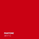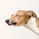4768 views
4768
This is my code for something Trading View already gives us -- Pivot Points .
It's not really an indicator in itself, although when weekly highs or lows are broken it seems to be a big deal. I wrote this so I'd have something to play with.

Here’s one way to access previous values:
valuewhen(change(high_series) != 0, high_series, 1)
```study(title="Pivot Points HL", shorttitle="Pivots H/L", overlay=true)

//The length defines how many periods a high or low must hold to be a "relevant pivot"
len = input(14, minval=1, title="Length")

//define a series that is equal to the highest value over the last length
highest_high = highest(high, len)
//if that high holds for the full length return the value, else na
highest_dev = dev(highest_high, len) ? na : highest_high
//shift the series to where it started
high_pivot = highest_dev[-len+1]
//create a series which is equal to the last pivot
high_series = fixnan(high_pivot)

//repeated for lows
lowest_low = lowest(low, len)
lowest_dev = dev(lowest_low, len) ? na : lowest_low
low_pivot = lowest_dev[-len+1]
low_series = fixnan(low_pivot)

plot(high_series, color=black, linewidth=2)
plot(low_series, color=black, linewidth=2)
//plot(high_pivot, color=black, style=circles, linewidth=4)
//plot(low_pivot, color=black, style=circles, linewidth=4)```Thank you, when applying the alert , Does it trigger when it hits the price or when it hits the line and how do I set it up in a way that it will trigger when it hits the price of the HH , LL, HL, LH. Thank you once again